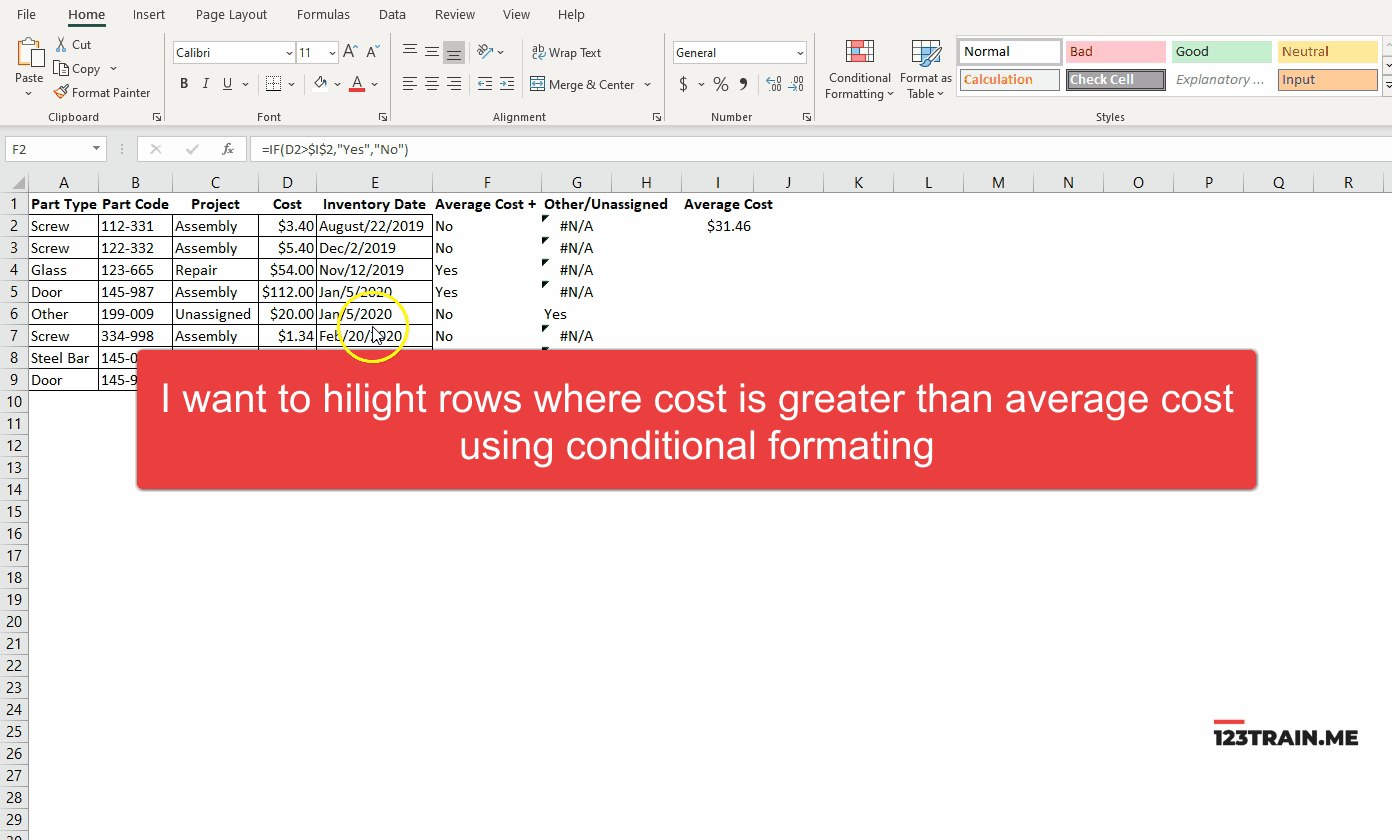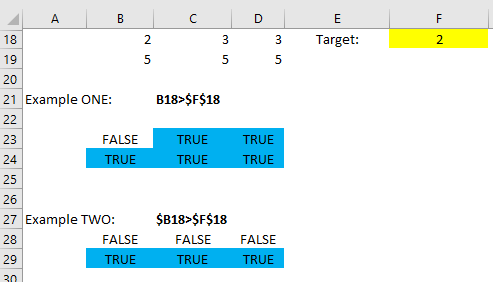top of page
Search

# Conditional FormattingCells can be formatted automatically based if a formula statement is TRUE.

1. Select the range of cells where the condition will apply.

2. On the Home tab select "Conditional Format" ribbon. Some of the most common formulas for conditional format are already pre set.

3. To create a custom formula select "Use a formula to determine which cells to format".

4. When typing a formula keep in mind that formula will evaluate for every cell.

For Example:Highlighted value is my target. The row with number values is the row which I attempt to evaluate. I want the cells to appear blue if the condition is true.

If I typed in formula in example one, all but the first cell would appear as blue. This is because in excel logic it will evaluate next cell by shifting all values to the right. My target stays the same because it is locked. However, B18 becomes C18 and therefore statement becomes TRUE.

In the Example TWO, I lock columns. Therefore when excel shifts to the next cell it is reminded it cannot shift columns. This means that value is still being evaluated as B18 and therefore statement is FALSE.

6 views

See All
bottom of page